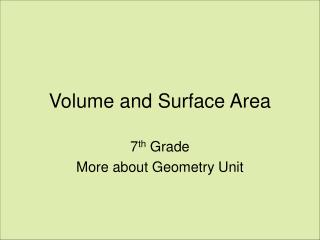DownloadDownload PresentationVolume and Surface Area

# Volume and Surface Area

Télécharger la présentation## Volume and Surface Area

- - - - - - - - - - - - - - - - - - - - - - - - - - - E N D - - - - - - - - - - - - - - - - - - - - - - - - - - -
##### Presentation Transcript

2. Definition • Surface Area – is the total number of unit squares used to cover a 3-D surface.

3. Find the SA of a Rectangular Solid A rectangular solid has 6 faces. They are: Front • Top • Bottom • Front • Back • Right Side • Left Side Top Right Side We can only see 3 faces at any one time. Which of the 6 sides are the same? • Top and Bottom • Front and Back • Right Side and Left Side

4. Surface Area of a Rectangular Solid We know that Each face is a rectangle. and the Formula for finding the area of a rectangle is: A = lw Front Top Right Side Steps: Find: Area of Top Area of Front Area of Right Side Find the sum of the areas Multiply the sum by 2. The answer you get is the surface area of the rectangular solid.

5. Find the Surface Area of the following: Find the Area of each face: 12 m Top 5 m A = 12 m x 5 m = 60 m2 Top Front Right Side 8 m Front 8 m A = 12 m x 8 m = 96 m2 5 m 12 m 12 m Right Side Sum = 60 m2 + 96 m2 + 40 m2 = 196 m2 A = 8 m x 5 m = 40 m2 8 m Multiply sum by 2 = 196 m2 x 2 = 392 m2 5 m The surface area = 392 m2

6. Find the Surface Area Area of Top = 6 cm x 4 cm = 24 cm2 Area of Front = 14 cm x 6 cm = 84 cm2 24 m2 Area of Right Side = 14 cm x 4 cm = 56 cm2 Find the sum of the areas: 56 m2 84 m2 14 cm 24 cm2 + 84 cm2 + 56 cm2 = 164 cm2 Multiply the sum by 2: 4 cm 164 cm2 x 2 = 328 cm2 6 cm The surface area of this rectangular solid is 328 cm2.

7. Nets • A net is all the surfaces of a rectangular solid laid out flat. Back 8 cm Top Left Side Top Right Side 5 cm 8 cm Right Side Front 8 cm Front 8 cm 5 cm 10 cm Bottom 5 cm 10 cm

8. Find the Surface Area using nets. Top Back 8 cm Right Side Front 8 cm Left Side Top Right Side 5 cm 5 cm 8 cm 10 cm Front 8 cm Each surface is a rectangle. A = lw 80 80 5 cm Bottom Find the area of each surface. Which surfaces are the same? Find the Total Surface Area. 40 10 cm 50 50 40 What is the Surface Area of the Rectangular solid? 340 cm2

9. 4cm 3cm 10cm 10cm 3cm Volumes Of Rectangular Prisms. Look at the rectangular prism below: We must first calculate the area of the base of the prism: The base is a rectangle measuring 10cm by 3cm:

10. 4cm 3cm 10cm 10cm 3cm Area of a rectangle = length x width Area = 10 x 3 Area = 30cm2 We now know we can place 30 centimetre squares on the base of the prism. But we can also place 30 cubic centimetres on the base:

11. 4cm 3cm 10cm We have now got to find how many layers of 1cm cubes we can place in the prism: We can fit in 4 layers. Volume = 30 x 4 Volume = 120cm3 That means that we can place 120 of our cubes measuring a centimetre in all directions inside our prism.

12. 4cm 3cm 10cm We have found that the volume of the cuboid is given by: Volume = 10 x 3 x 4 = 120cm3 This gives us our formula for the volume of a prism: Volume = Area of Base x Height V=AbaseH for short.

13. 6cm 4cm The Volume Of A Cylinder. Consider the cylinder below: It has a height of 6cm . What is the size of the radius ? 2cm Volume = Abasex height What shape is the base? Circle Calculate the area of the circle: A = r 2 A = 3.14 x 2 x 2 The formula for the volume of a cylinder is: V =Abase x height = r 2 h r = radius h = height A = 12.56 cm2 Calculate the volume: V = r 2 x h V = 12.56 x 6 V = 75.36 cm3

14. 5cm 8cm 5cm The Volume Of A Triangular Prism. Consider the triangular prism below: Volume = AbasexHeight What shape is the base ? Triangle. Calculate the area of the triangle: A = (base x height)/2 A = (5 x 5)/2 A = 12.5cm2 Calculate the volume: Volume = Abasex Height The formula for the volume of a triangular prism is : V = ½ b h h B= base h = height h = height V = 12.5 x 8 V = 100 cm3

15. (1) (2) 14cm 4m 5m 16cm 3m (3) 8m 12cm 6cm What Goes In The Box ? 2 Calculate the volume of the shapes below: 2813.4cm3 30m3 288cm3